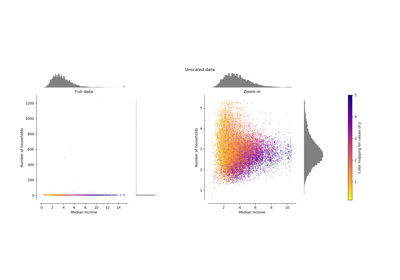# sklearn.preprocessing.RobustScaler¶

class sklearn.preprocessing.RobustScaler(with_centering=True, with_scaling=True, quantile_range=(25.0, 75.0), copy=True)[source]

Scale features using statistics that are robust to outliers.

This Scaler removes the median and scales the data according to the quantile range (defaults to IQR: Interquartile Range). The IQR is the range between the 1st quartile (25th quantile) and the 3rd quartile (75th quantile).

Centering and scaling happen independently on each feature by computing the relevant statistics on the samples in the training set. Median and interquartile range are then stored to be used on later data using the transform method.

Standardization of a dataset is a common requirement for many machine learning estimators. Typically this is done by removing the mean and scaling to unit variance. However, outliers can often influence the sample mean / variance in a negative way. In such cases, the median and the interquartile range often give better results.

New in version 0.17.

Read more in the User Guide.

Parameters
with_centeringboolean, True by default

If True, center the data before scaling. This will cause transform to raise an exception when attempted on sparse matrices, because centering them entails building a dense matrix which in common use cases is likely to be too large to fit in memory.

with_scalingboolean, True by default

If True, scale the data to interquartile range.

quantile_rangetuple (q_min, q_max), 0.0 < q_min < q_max < 100.0

Default: (25.0, 75.0) = (1st quantile, 3rd quantile) = IQR Quantile range used to calculate scale_.

New in version 0.18.

copyboolean, optional, default is True

If False, try to avoid a copy and do inplace scaling instead. This is not guaranteed to always work inplace; e.g. if the data is not a NumPy array or scipy.sparse CSR matrix, a copy may still be returned.

Attributes
center_array of floats

The median value for each feature in the training set.

scale_array of floats

The (scaled) interquartile range for each feature in the training set.

New in version 0.17: scale_ attribute.

robust_scale

Equivalent function without the estimator API.

sklearn.decomposition.PCA

Further removes the linear correlation across features with ‘whiten=True’.

Notes

For a comparison of the different scalers, transformers, and normalizers, see examples/preprocessing/plot_all_scaling.py.

Examples

>>> from sklearn.preprocessing import RobustScaler
>>> X = [[ 1., -2.,  2.],
...      [ -2.,  1.,  3.],
...      [ 4.,  1., -2.]]
>>> transformer = RobustScaler().fit(X)
>>> transformer
RobustScaler()
>>> transformer.transform(X)
array([[ 0. , -2. ,  0. ],
[-1. ,  0. ,  0.4],
[ 1. ,  0. , -1.6]])


Methods

 fit(self, X[, y]) Compute the median and quantiles to be used for scaling. fit_transform(self, X[, y]) Fit to data, then transform it. get_params(self[, deep]) Get parameters for this estimator. inverse_transform(self, X) Scale back the data to the original representation set_params(self, \*\*params) Set the parameters of this estimator. transform(self, X) Center and scale the data.
__init__(self, with_centering=True, with_scaling=True, quantile_range=(25.0, 75.0), copy=True)[source]

Initialize self. See help(type(self)) for accurate signature.

fit(self, X, y=None)[source]

Compute the median and quantiles to be used for scaling.

Parameters
Xarray-like, shape [n_samples, n_features]

The data used to compute the median and quantiles used for later scaling along the features axis.

fit_transform(self, X, y=None, **fit_params)[source]

Fit to data, then transform it.

Fits transformer to X and y with optional parameters fit_params and returns a transformed version of X.

Parameters
Xnumpy array of shape [n_samples, n_features]

Training set.

ynumpy array of shape [n_samples]

Target values.

**fit_paramsdict

Returns
X_newnumpy array of shape [n_samples, n_features_new]

Transformed array.

get_params(self, deep=True)[source]

Get parameters for this estimator.

Parameters
deepbool, default=True

If True, will return the parameters for this estimator and contained subobjects that are estimators.

Returns
paramsmapping of string to any

Parameter names mapped to their values.

inverse_transform(self, X)[source]

Scale back the data to the original representation

Parameters
Xarray-like

The data used to scale along the specified axis.

set_params(self, **params)[source]

Set the parameters of this estimator.

The method works on simple estimators as well as on nested objects (such as pipelines). The latter have parameters of the form <component>__<parameter> so that it’s possible to update each component of a nested object.

Parameters
**paramsdict

Estimator parameters.

Returns
selfobject

Estimator instance.

transform(self, X)[source]

Center and scale the data.

Parameters
X{array-like, sparse matrix}

The data used to scale along the specified axis.

## Examples using sklearn.preprocessing.RobustScaler¶